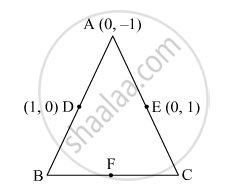Advertisement Remove all ads

# In δ Abc , the Coordinates of Vertex a Are (0, - 1) and D (1,0) and E(0,10) Respectively the Mid-points of the Sides Ab and Ac . If F is the Mid-points of the Side Bc , Find the Area of δ Def. - Mathematics

Answer in Brief

In  $∆$ ABC , the coordinates of vertex A are (0, - 1) and D (1,0) and E(0,10)  respectively the mid-points of the sides AB and AC . If F is the mid-points of the side BC , find the area of $∆$ DEF.

Advertisement Remove all ads

#### SolutionLet the coordinates of B and C be  $\left( x_2 , y_2 \right)$  and $\left( x_3 , y_3 \right)$ , respectively.

D is the midpoint of AB.
So,

$\left( 1, 0 \right) = \left( \frac{x_2 + 0}{2}, \frac{y_2 - 1}{2} \right)$

$\Rightarrow 1 = \frac{x_2}{2} \text { and } 0 = \frac{y_2 - 1}{2}$

$\Rightarrow x_2 = 2 \text{ and } y_2 = 1$

Thus, the coordinates of B are (2, 1).

Similarly, E is the midpoint of AC.

So,

$\left( 0, 1 \right) = \left( \frac{x_3 + 0}{2}, \frac{y_3 - 1}{2} \right)$

$\Rightarrow 0 = \frac{x_3}{2} \text{ and } 1 = \frac{y_3 - 1}{2}$

$\Rightarrow x_3 = 0 \text{ and } y_3 = 3$

Thus, the coordinates of C are (0, 3).
Also, F is the midpoint of BC. So, its coordinates are  $\left( \frac{2 + 0}{2}, \frac{1 + 3}{2} \right) = \left( 1, 2 \right)$

Now,
Area of a triangle =  $\frac{1}{2}\left[ x_1 \left( y_2 - y_3 \right) + x_2 \left( y_3 - y_1 \right) + x_3 \left( y_1 - y_2 \right) \right]$
Thus, the area of $∆$ ABC is

$\frac{1}{2}\left[ 0\left( 1 - 3 \right) + 2\left( 3 + 1 \right) + 0\left( - 1 - 1 \right) \right]$

$= \frac{1}{2} \times 8$

$= 4 \text{ square units }$

And the area of  $∆$  DEF is

$\frac{1}{2}\left[ 1\left( 1 - 2 \right) + 0\left( 2 - 0 \right) + 1\left( 0 - 1 \right) \right]$

$= \frac{1}{2} \times \left( - 2 \right)$

$= 1 \text{ square unit } \left( \text{ Taking the numerical value, as the area cannot be negative } \right)$

Is there an error in this question or solution?
Advertisement Remove all ads

#### APPEARS IN

RD Sharma Class 10 Maths
Chapter 6 Co-Ordinate Geometry
Exercise 6.5 | Q 7 | Page 54
Advertisement Remove all ads

#### Video TutorialsVIEW ALL 

Advertisement Remove all ads
Share
Notifications

View all notifications

Forgot password?